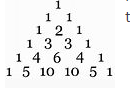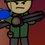# Pascals Triangle; who made up the combination pattern?Here is the pascals triangle. It was invented by the Chinese, but since we are technically Europeans, we know it as Pascal's Triangle because we first knew it thanks to Pascal. But one thing I really don't understand, is why they notation combinations as $\frac{n!}{r!(n-r)!}$, and they just put it in to use in the triangle. like, seriously. I need help.Note by Odin Wang
10 months, 2 weeks ago

This discussion board is a place to discuss our Daily Challenges and the math and science related to those challenges. Explanations are more than just a solution — they should explain the steps and thinking strategies that you used to obtain the solution. Comments should further the discussion of math and science.

When posting on Brilliant:

• Use the emojis to react to an explanation, whether you're congratulating a job well done , or just really confused .
• Ask specific questions about the challenge or the steps in somebody's explanation. Well-posed questions can add a lot to the discussion, but posting "I don't understand!" doesn't help anyone.
• Try to contribute something new to the discussion, whether it is an extension, generalization or other idea related to the challenge.
• Stay on topic — we're all here to learn more about math and science, not to hear about your favorite get-rich-quick scheme or current world events.

MarkdownAppears as
*italics* or _italics_ italics
**bold** or __bold__ bold
- bulleted- list
• bulleted
• list
1. numbered2. list
1. numbered
2. list
Note: you must add a full line of space before and after lists for them to show up correctly
paragraph 1paragraph 2

paragraph 1

paragraph 2

[example link](https://brilliant.org)example link
> This is a quote
This is a quote
    # I indented these lines
# 4 spaces, and now they show
# up as a code block.

print "hello world"
# I indented these lines
# 4 spaces, and now they show
# up as a code block.

print "hello world"
MathAppears as
Remember to wrap math in $$ ... $$ or $ ... $ to ensure proper formatting.
2 \times 3 $2 \times 3$
2^{34} $2^{34}$
a_{i-1} $a_{i-1}$
\frac{2}{3} $\frac{2}{3}$
\sqrt{2} $\sqrt{2}$
\sum_{i=1}^3 $\sum_{i=1}^3$
\sin \theta $\sin \theta$
\boxed{123} $\boxed{123}$

## Comments

Sort by:

Top Newest

Because in the nth line the kth element is equal to $\dbinom{n}{k}$. And: \begin{aligned} \dbinom{n}{k}&=\dbinom{n-1}{k-1}+\dbinom{n-1}{k}\\ \cfrac{n!}{k!(n-k)!}&=\cfrac{(n-1)!}{(k-1)!(n-k)!}+\cfrac{(n-1)!}{k!(n-k-1)!}&\left \| \times\cfrac{(k-1)!(n-k-1)!}{(n-1)!}\right .\\ \cfrac{n}{k(n-k)}&=\cfrac{1}{(n-k)}+\cfrac{1}{k}\\ \cfrac{n}{k(n-k)}&=\cfrac{k}{k(n-k)}+\cfrac{n-k}{k(n-k)}\\ \cfrac{n}{k(n-k)}&=\cfrac{n}{k(n-k)} \end{aligned} So if this is true for the first elements, then it will be always true.

- 10 months, 2 weeks ago

Log in to reply

I see

- 10 months, 2 weeks ago

Log in to reply

do you want to know what the triangle can be used for?

- 10 months, 2 weeks ago

Log in to reply

No thanks, I know the path trick where the Pascal’s triangle is useful.

- 10 months, 2 weeks ago

Log in to reply

# MathHistory

- 10 months, 2 weeks ago

Log in to reply

In India it is known as Meru Prastara

- 10 months, 1 week ago

Log in to reply

Everywhere it is different unless it isn't

- 10 months, 1 week ago

Log in to reply

×

Problem Loading...

Note Loading...

Set Loading...